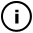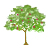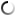# Thursday, February 25, 2010

## Collecting paper corners

int maxDepth = 35;
int currentDepth = 0;
int justStart = 1;
float tangle;

int stage = 2;
float angle = 0;
float tx, ty;

color cp;
float value;

int translationX;
int translationY;
float rotation;

int x1,y1,x2,y2,x1f,y1f,x2f,y2f;

void setup()
{
background(255);
size(800,400);
}

void drawShard() {

int limit = depth+int(random(0,10));

// the red component below is 254
// at most because we test the value
// 255 for collisions
stroke(random(0,254),random(0,255),random(0,255),99);

for(int i = 0; i < limit; i++)
{

x1 = i+translationX;
y1 = translationY+depth;

x2 = x1;
y2 = y1-depth;

x1f = int(x1 * cos(rotation) - y1 * sin(rotation)) ;
y1f = int(x1 * sin(rotation) + y1 * cos(rotation));

x2f = int(x2 * cos(rotation) - y2 * sin(rotation));
y2f = int(x2 * sin(rotation) + y2 * cos(rotation));

//println(i);
//println(start);
//color cp = pixels[i+ start*width];
x1f = constrain(x1f,0,width-1);
y1f = constrain(y1f,0,height-1);
x2f = constrain(x2f,0,width-1);
y2f = constrain(y2f,0,height-1);

// for each line, we test collision
// with a previously-drawn shape
// at beginning, end and middle

if (red(get(x1f, y1f)) != 255) return;

if (red(get(int((x1f+x2f)/2), int((y1f+y2f)/2))) != 255) return;

if (red(get(x2f, y2f)) != 255) return;

}

for(int i = 0; i < limit; i++)
{

x1 = i+translationX;
y1 = translationY+depth;

x2 = x1;
y2 = y1-depth;

// we perform the rotation transformation here
// we cant use the usual processing
// matrix way of doing things because when we test for previously-painted pixels we have to pass the transformed coordinates
x1f = int(x1 * cos(rotation) - y1 * sin(rotation)) ;
y1f = int(x1 * sin(rotation) + y1 * cos(rotation));

x2f = int(x2 * cos(rotation) - y2 * sin(rotation));
y2f = int(x2 * sin(rotation) + y2 * cos(rotation));

if (depth != 0 ) {

line(x1f,y1f,x2f,y2f);
}
//println(int(start));
//cp = get(int(l), int(start));
//value = red(cp);
//println(value);
//if (value != 255) return;

}

}

void draw()
{
//translate(random(0,width),random(0,height));
//rotate(random(-1,1));

translationX = int(random(0,width));
translationY = int(random(0,height));
rotation = random(-1,1);
drawShard();

}

void mousePressed()
{
background(255);
}

## infosubmitted by: DARYL_Gamma
views: 610
This sketch takes quite some time to render (5 minutes or so). It tries to randomly fill empty spots with shapes of decreasing dimension. Most overlaps are avoided, but some small collisions happen.This sketch has a parent
Tags: slices, collision, filling

## commentsloading...